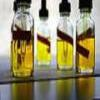#### You may also like### Mathematical Issues for Chemists

A brief outline of the mathematical issues faced by chemistry students.### Reaction Rates

Explore the possibilities for reaction rates versus concentrations with this non-linear differential equation### Blood Buffers

Investigate the mathematics behind blood buffers and derive the form of a titration curve.

# Catalyse That!

##### Age 16 to 18 Challenge Level:

The rate of an enzyme-catalysed reaction depends on the temperature of its solution. The reaction activates at $20^\circ C$, at a rate of zero mol/l/min, and deactivates at $80^\circ C$. For each degree increase in temperature between $20^\circ C$ and $60^\circ C$ the rate of reaction increases by 0.1 mol/l/min and for each degree increment between $60^\circ C$ and $80^\circ C$ the rate reduces by 0.2 mol/l/min.
10 litres of the solution is prepared at a temperature of $20^\circ C$ and then placed immediately into an oven. Assume that the oven heats the solution at a fixed rate of $R^\circ C$ per minute. Consider these questions
1. What rate of heating would give rise to exactly 100 mol being catalysed?
2. For heating at a rate of 1 degree per minute, after how long will exactly 100 mol been catalysed?
3. (Hard numerical extension) What rate of heating would lead to exactly 100 mol being catalysed in the shortest time?
Consider an alternative problem: if the solution is prepared at boiling point: at what rate must 1 litre be cooled to end up with exactly 3 mol having been catalysed?

Hard extension: In reality, the temperature of the oven would probably be fixed and the rate of increase in temperature of the solution would be proportional to the difference in temperature between the oven and the solution. How far are you able to analyse this situation (note: you need to use differential equations)?

#### Notes and background

Whilst the chemistry of certain processes might seem reasonably straightforward, to implement reactions on an industrial scale requires very precise levels of timing, heating and so on. Since a human is unable carefully to watch a reaction progress in many cases, checking mechanisms must be automated. Scaling up reactions to large volumes can introduce many engineering complications not seen in the laboratory.

Furthermore, many chemical and biological reactions will naturally activate once certain temperatures are reached. Measuring a specific quantity of reactants in these cases might be tricky, and the amount taken out of the freezer, for example, might need to be smaller than the amount required for an experiment so as to take into account this (inevitable) continuous growth.

More realistically, heating does not typically occur at a fixed rate. Newton's Law of Cooling tells us that the rate of change in temperature is proportional to the difference in the temperature between the body and the heat source.